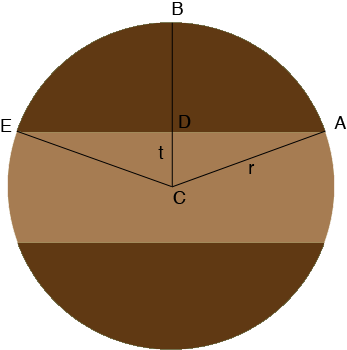SEARCH HOMEMath Central Quandaries & QueriesQuestion from James: I'm wondering if there's a simple way to calculate the area between two parallel chords of a circle equidistant from its diameter, or if I have the area, to find the distance between the two chords. Here's my "problem". You may have heard of the way of cutting a round cake so that it doesn't dry out - make two parallel cuts (chords) the length of the cake, take the middle piece, then push the two pieces together. So I know the area of a 12" cake, and I want say, exactly an eighth of the cake. How wide do I cut the centre piece? Now to get even more difficult, the next day I want another eighth from the centre. How wide do I cut the next pieces, and so on...? Thanks, JamesHi James,

I'm going to write an expression for the area of the "cap" $ABED$ as the area of the sector $CABE$ minus the area of the triangle $CAE.$The area of a sector of a circle of radius $r$ is $\large \frac12 \normalsize r^2 \theta$ where $\theta$ is the central angle measured in radians. In this case the central angle is the angle $ECA$ which is twice the angle $DCA.$ Since triangle $ADC$ is a right triangle

$\cos\left(\frac{\theta}{2}\right) = \frac{t}{r}$

and hence

$\frac{\theta}{2} = \cos^{-1}\left(\frac{t}{r}\right)$

and thus the area of the sector $CABE$ is

$r^2 \cos^{-1}\left(\frac{t}{r}\right).$

Since triangle $ADC$ is a right triangle Pythagoras Theorem tell us that the length of $DA$ is $\sqrt{r^2 - t^2}$ and hence the area of the triangle $CAE$ is

$t \sqrt{r^2 - t^2}$

and thus the area of the cap $ABED$ is

$r^2 \cos^{-1}\left(\frac{t}{r}\right) - t \sqrt{r^2 - t^2}$

and hence the area of the light brown slice you want to remove from the cake is

$\pi \; r^2 - 2 r^2 \cos^{-1}\left(\frac{t}{r}\right) + 2 t \sqrt{r^2 - t^2}.$

In the situation you describe with a 12 inch diameter cake from which you want to remove a centre slice with surface area one eighth of the cake you have

$\frac18 \pi \; \times 36 = \pi \; \times 36 - 2 \times 36 \; \cos^{-1}\left(\frac{t}{6}\right) + 2 t \sqrt{36 - t^2}$

and you want to solve for $t.$

This expression can be simplified somewhat to give the equation

$\frac{63}{4} \pi - 36 \; \cos^{-1}\left(\frac{t}{6}\right) + t \sqrt{36 - t^2}= 0$

but unfortunately this equation can't be solved for $t$ to give the "simple" solution you desire.

HarleyMath Central is supported by the University of Regina and the Imperial Oil Foundation.